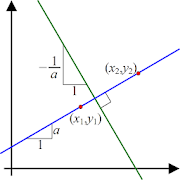# Slope and Linear Equation Calculator - Lumos Educational App Store2.61
Price -fREE
\$0

#### DESCRIPTION:

Easily calculate Slope and Linear Equation! - Save yourself time and effort - Calculate Slope - Calculate y-intercept - Determine Linear Equation - Clear results - Recalculate any number of times - Offline support

#### OVERVIEW:

Slope and Linear Equation Calculator is a free educational mobile app By .It helps students in grades HS practice the following standards HSF.IF.C.7.A.

This page not only allows students and teachers download Slope and Linear Equation Calculator but also find engaging Sample Questions, Videos, Pins, Worksheets, Books related to the following topics.

1. HSF.IF.C.7.A : Graph linear and quadratic functions and show intercepts, maxima, and minima.

HS

#### STANDARDS:

HSF.IF.C.7.A

Developer:

Software Version: 4

Category:

### RELATED APPS